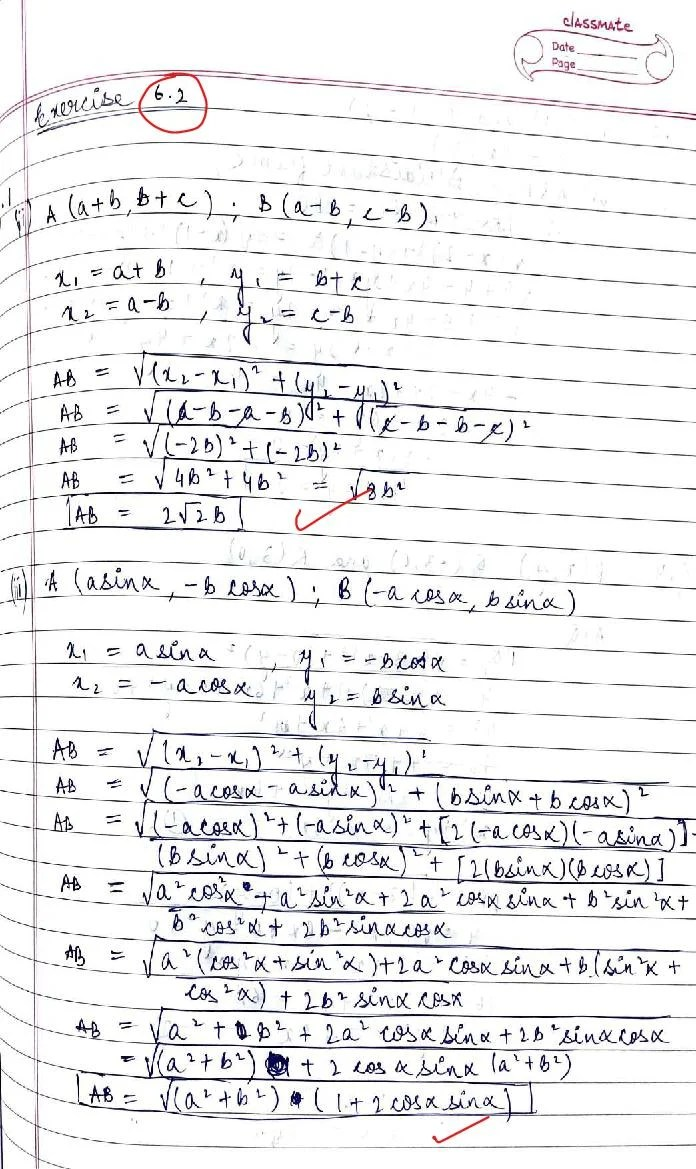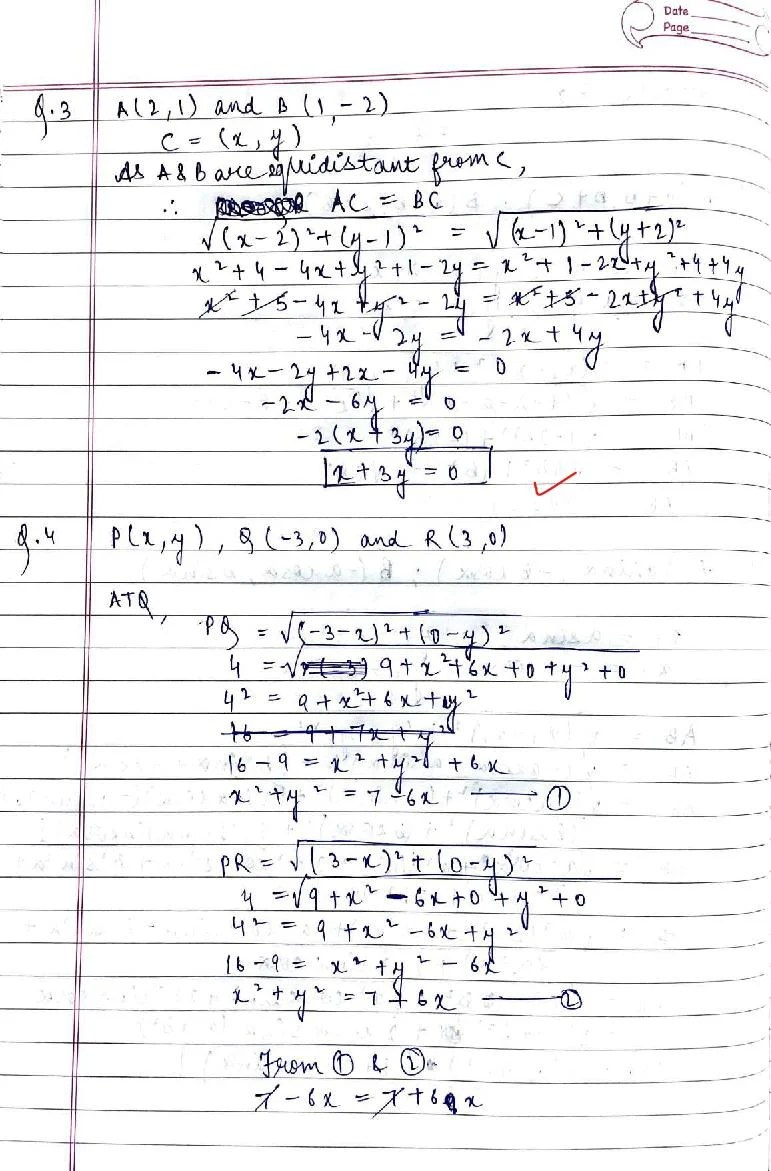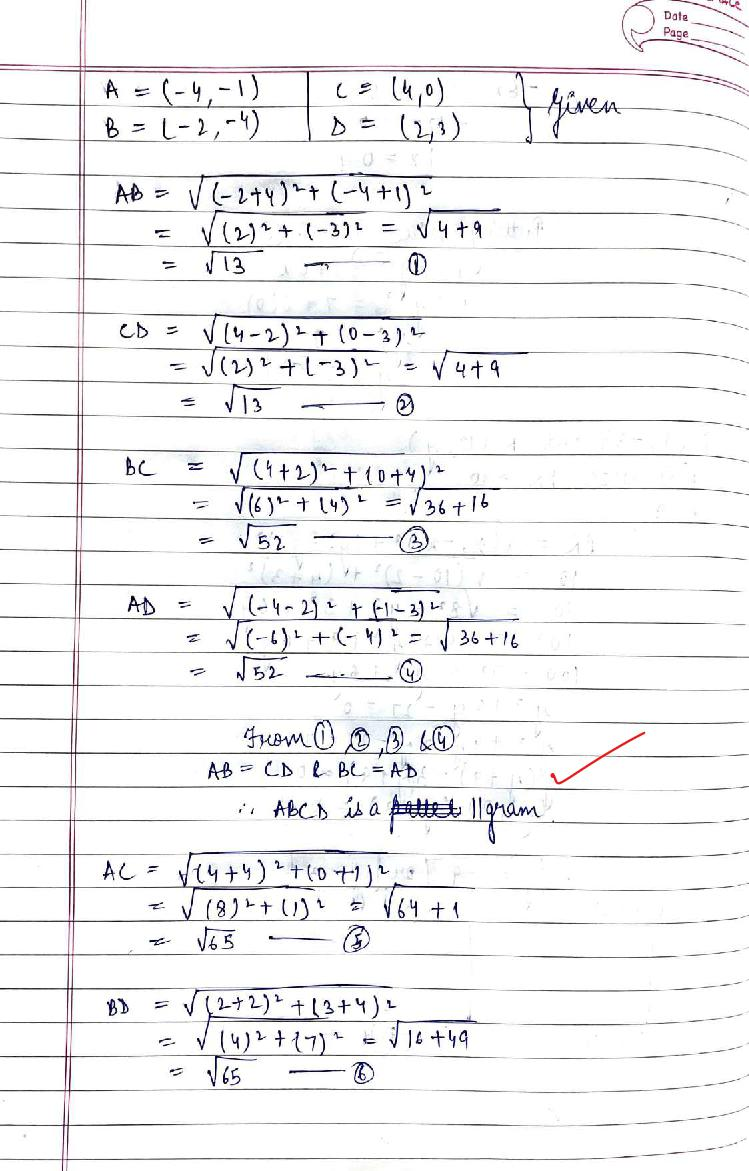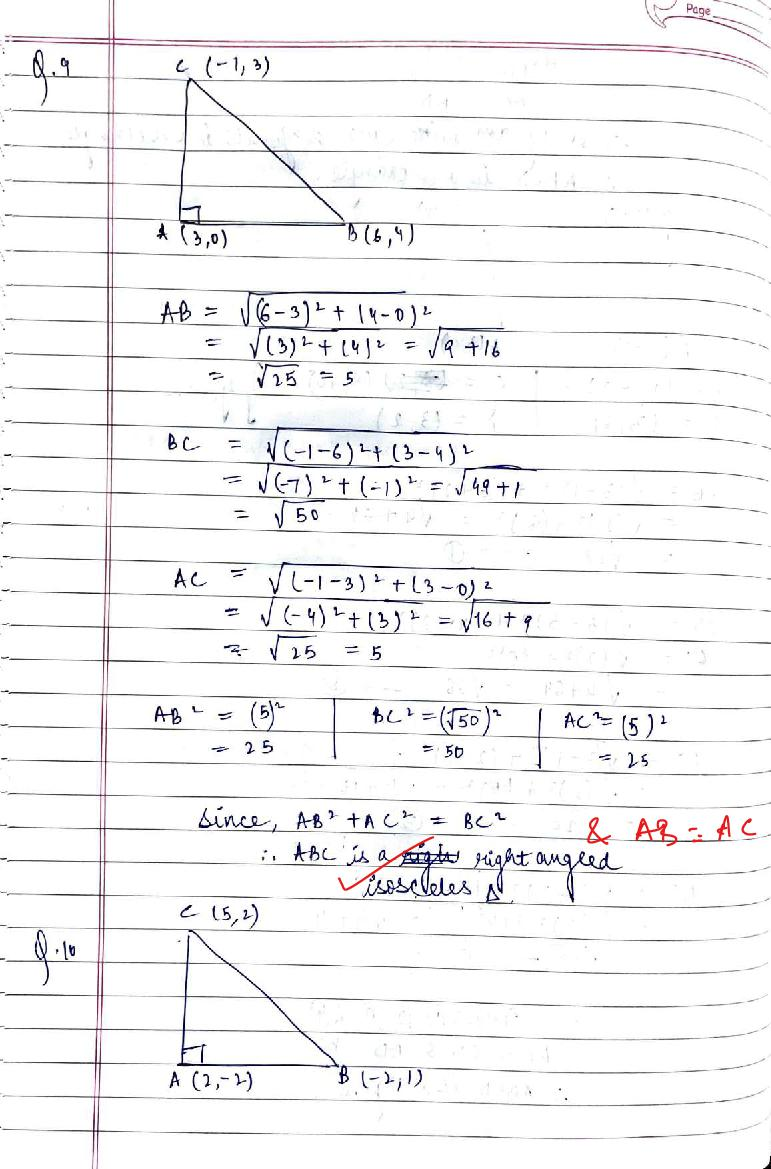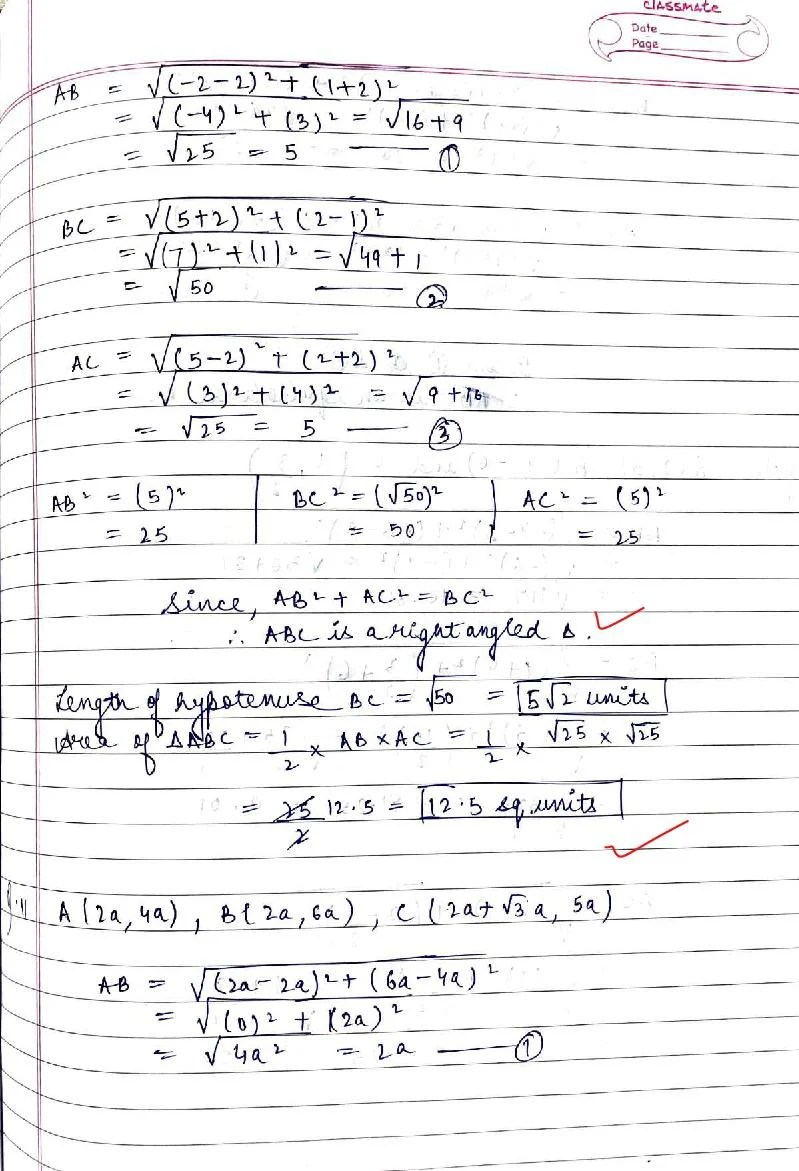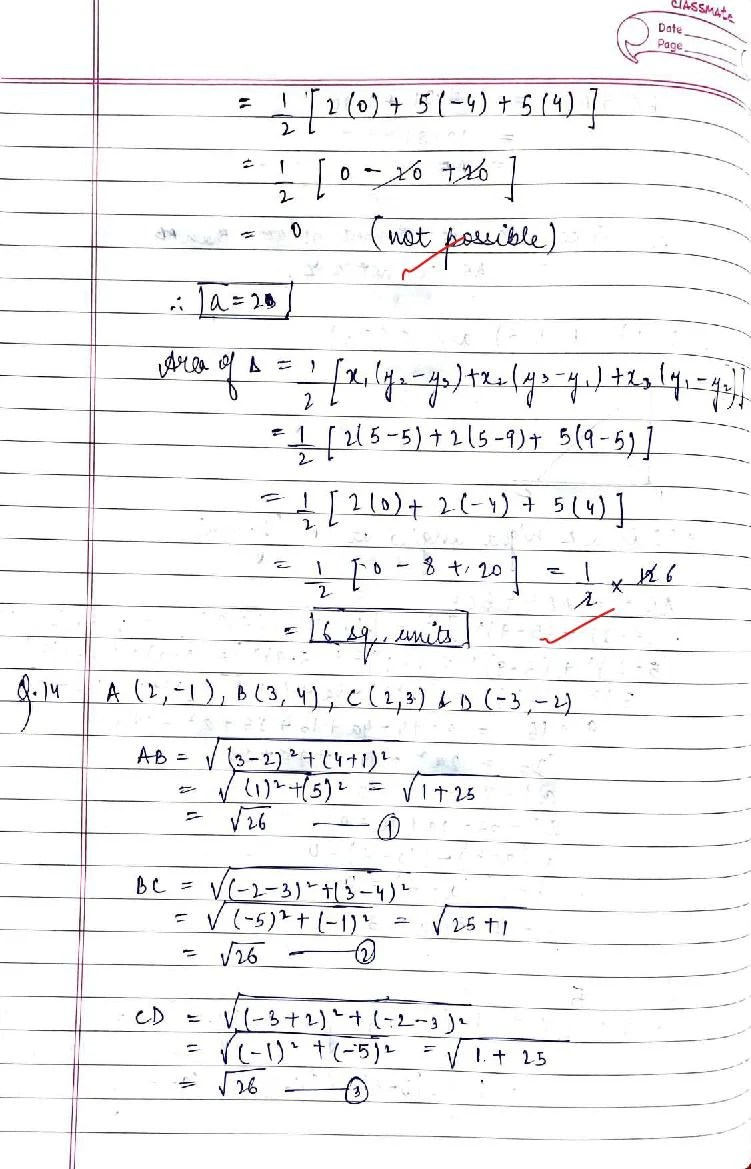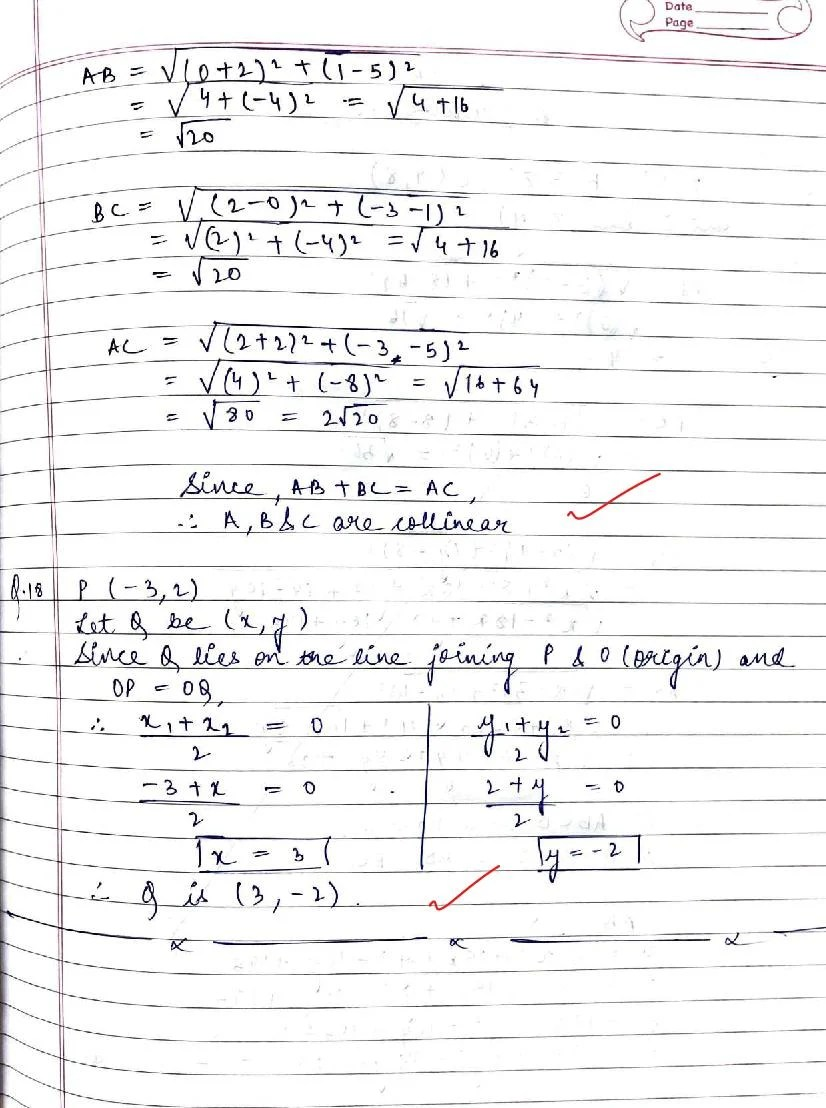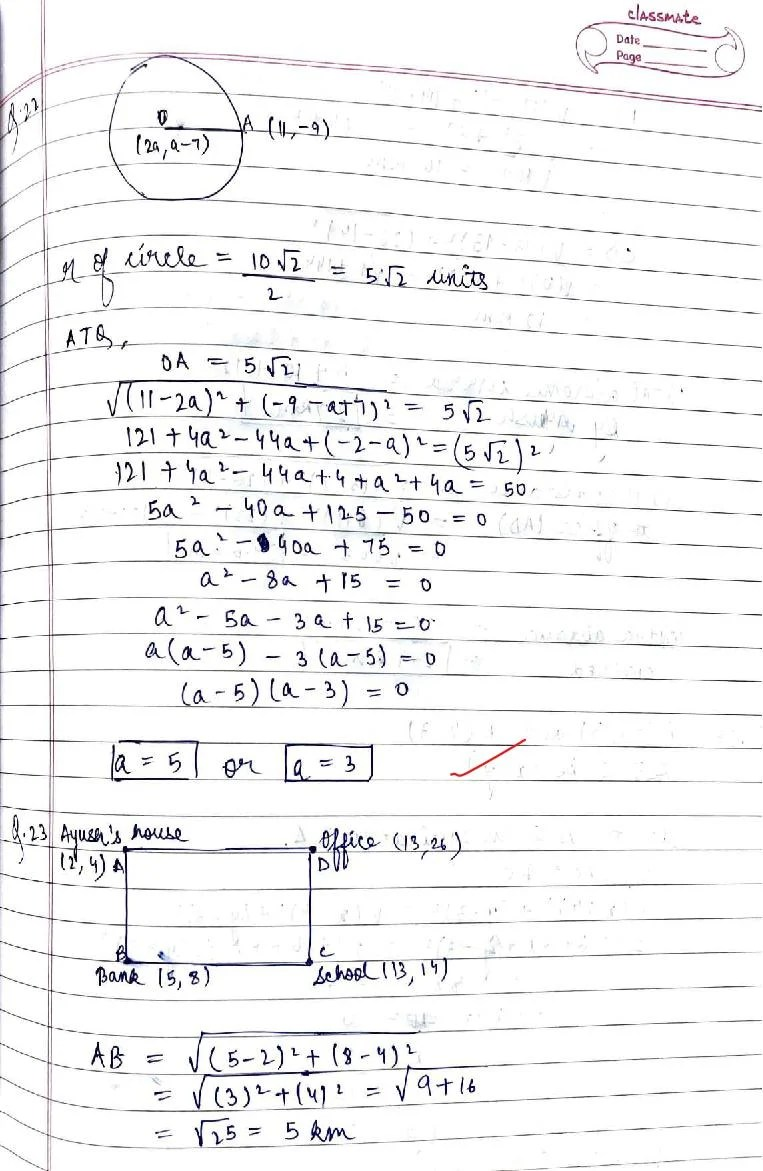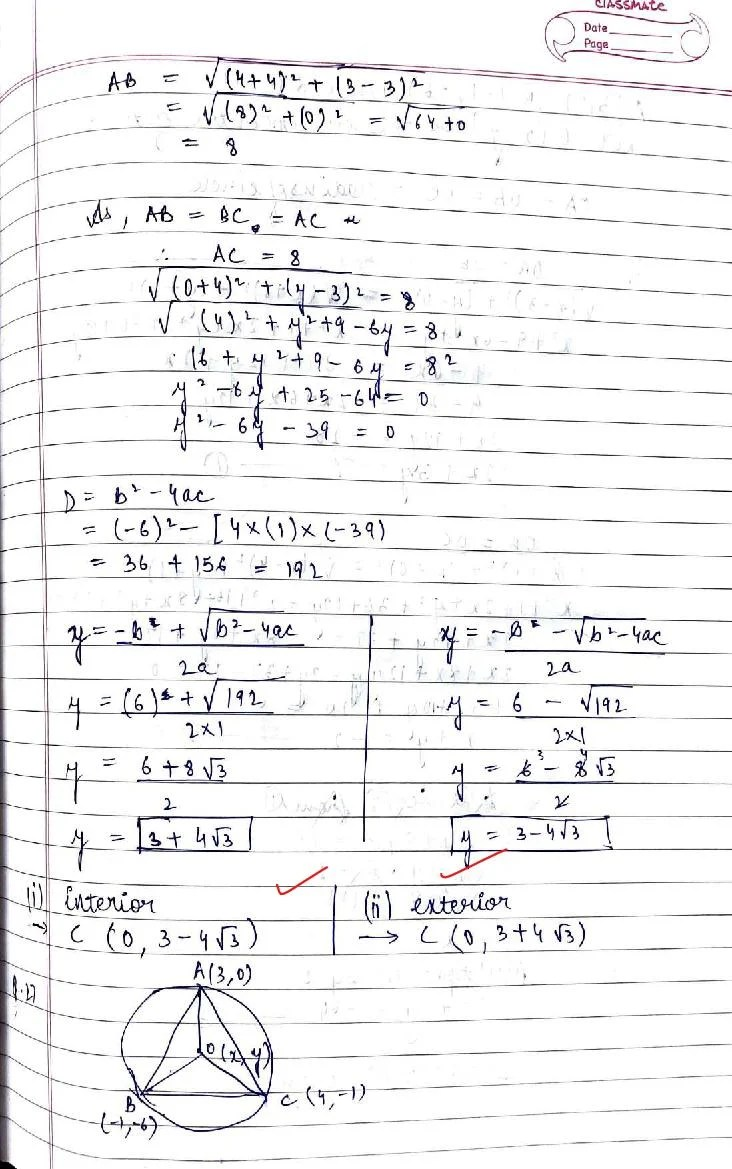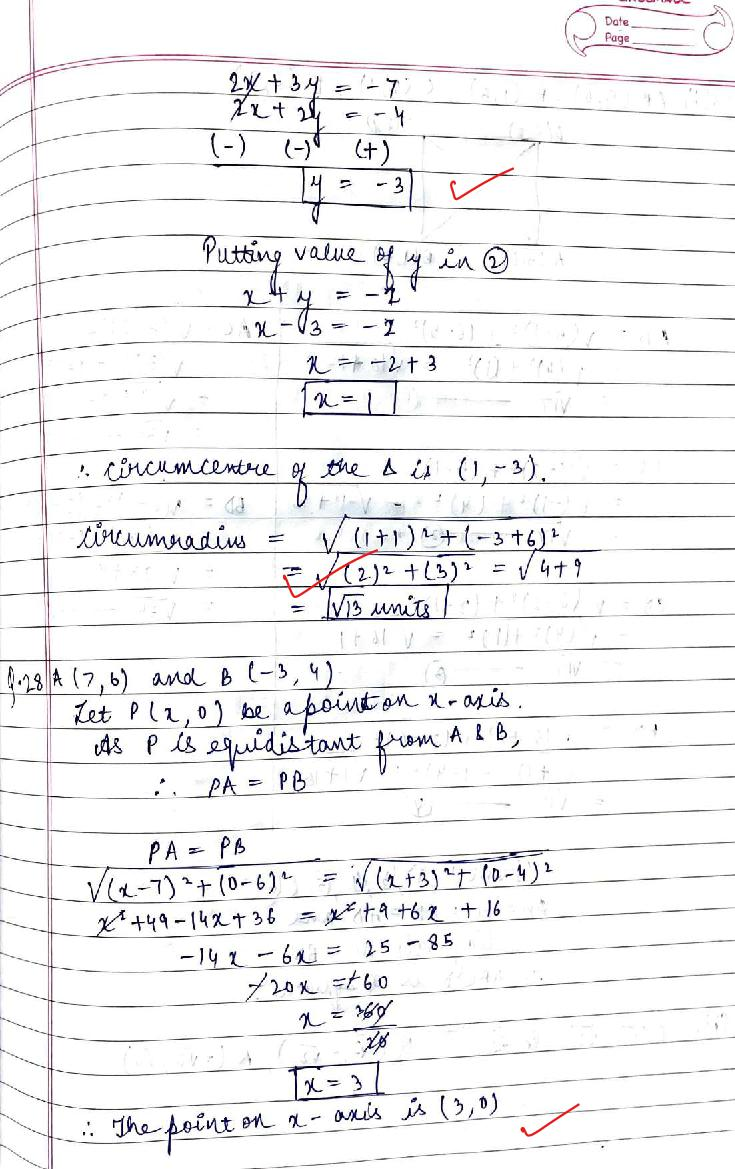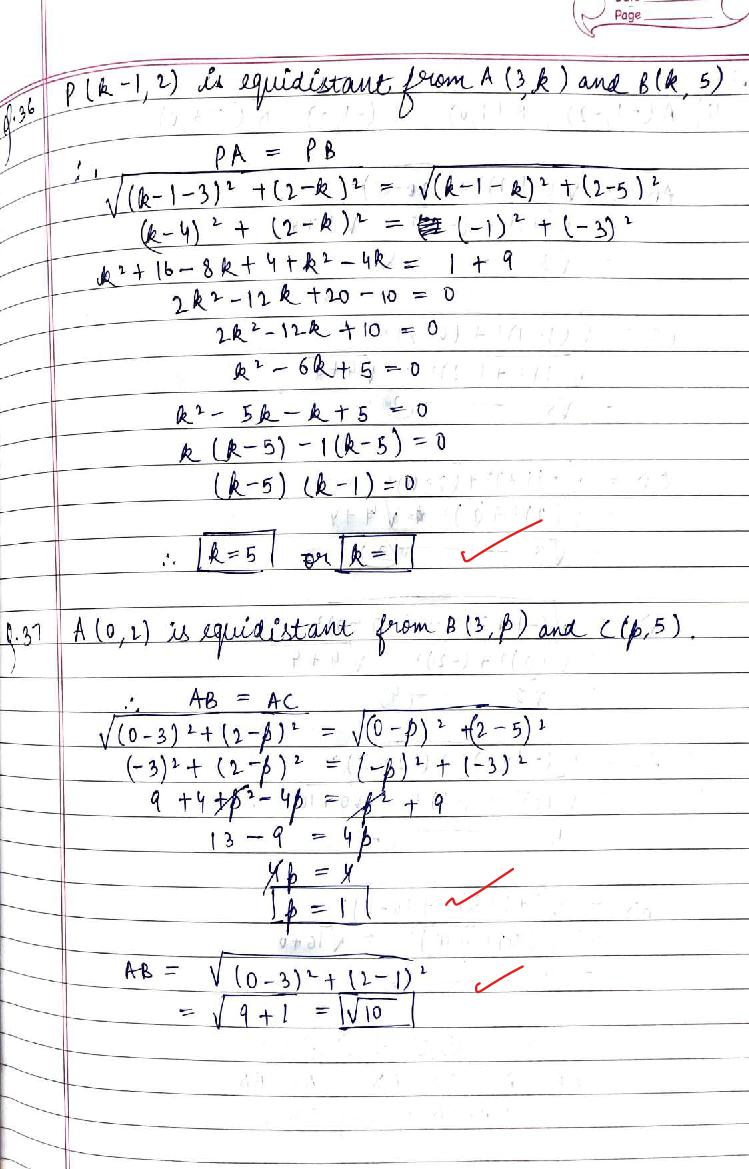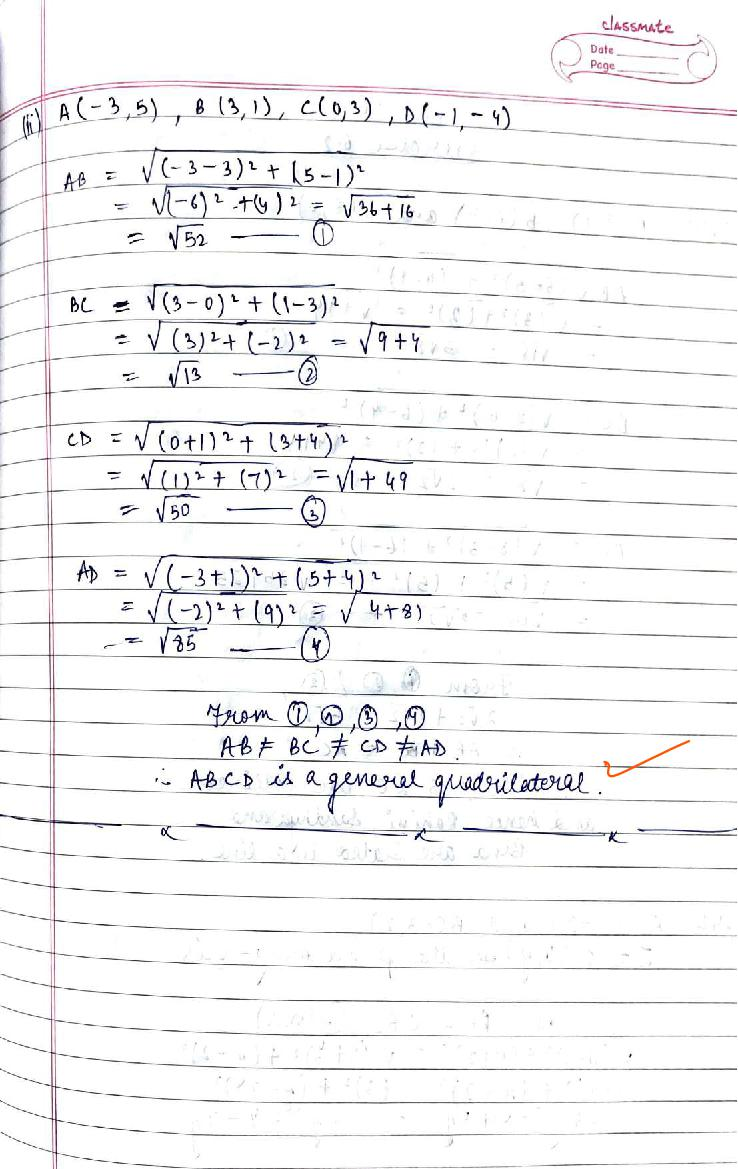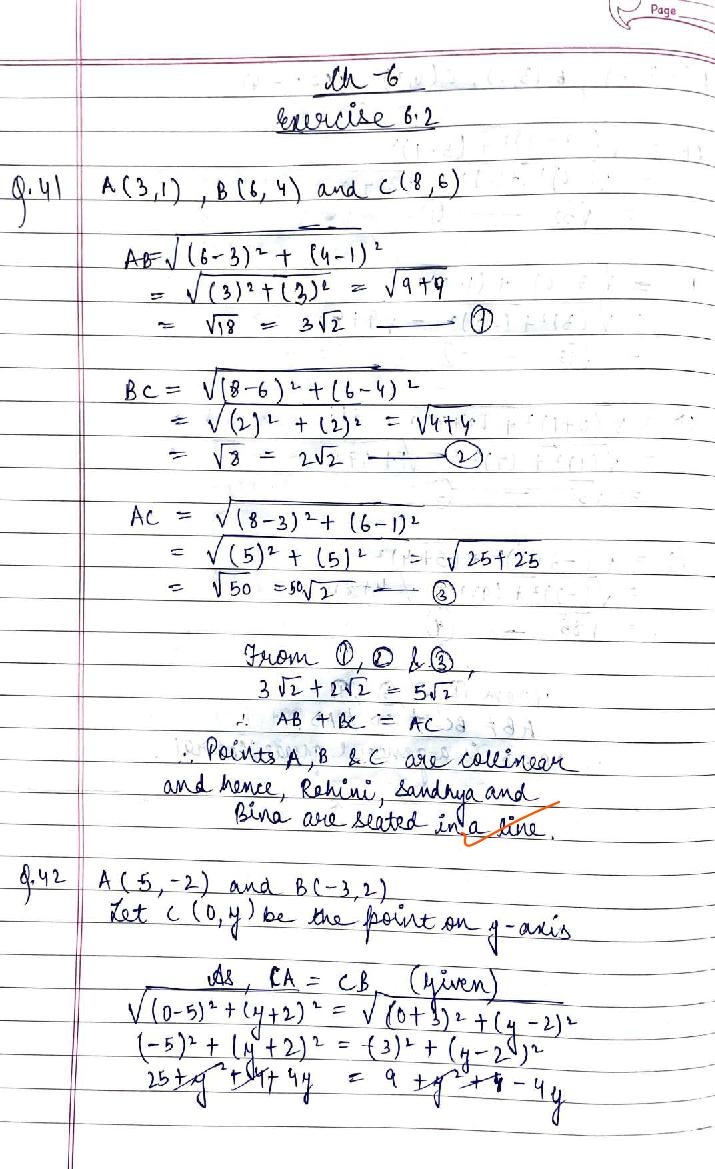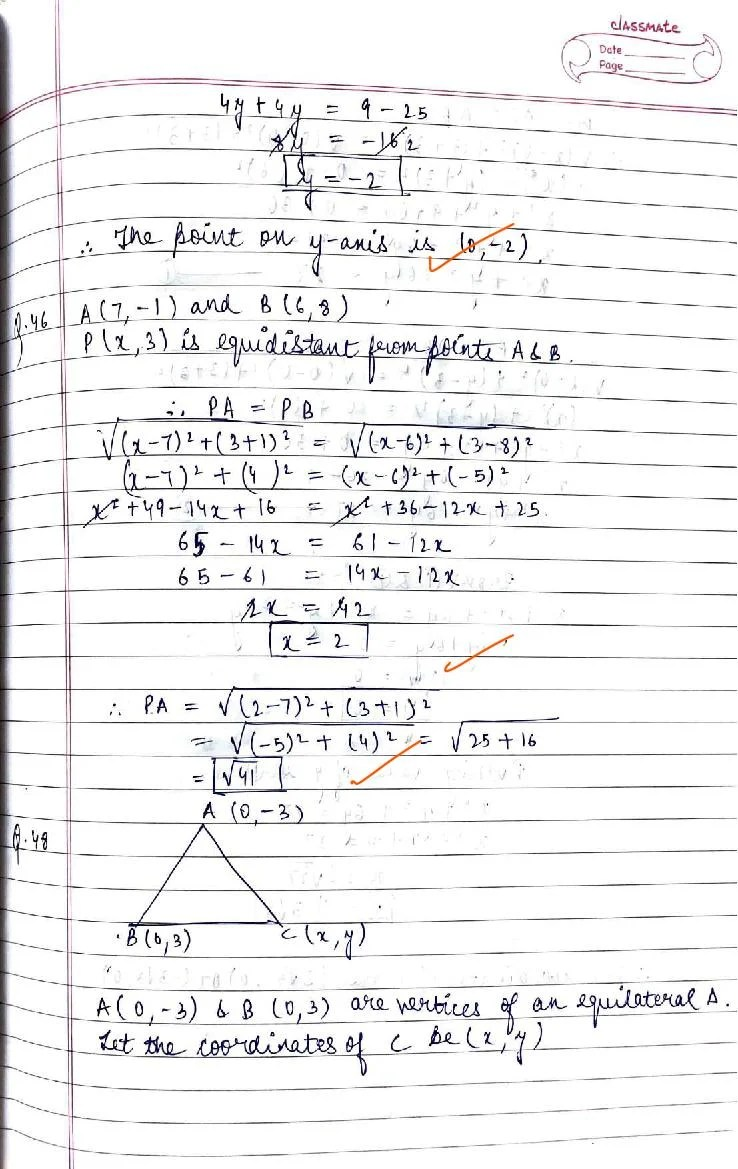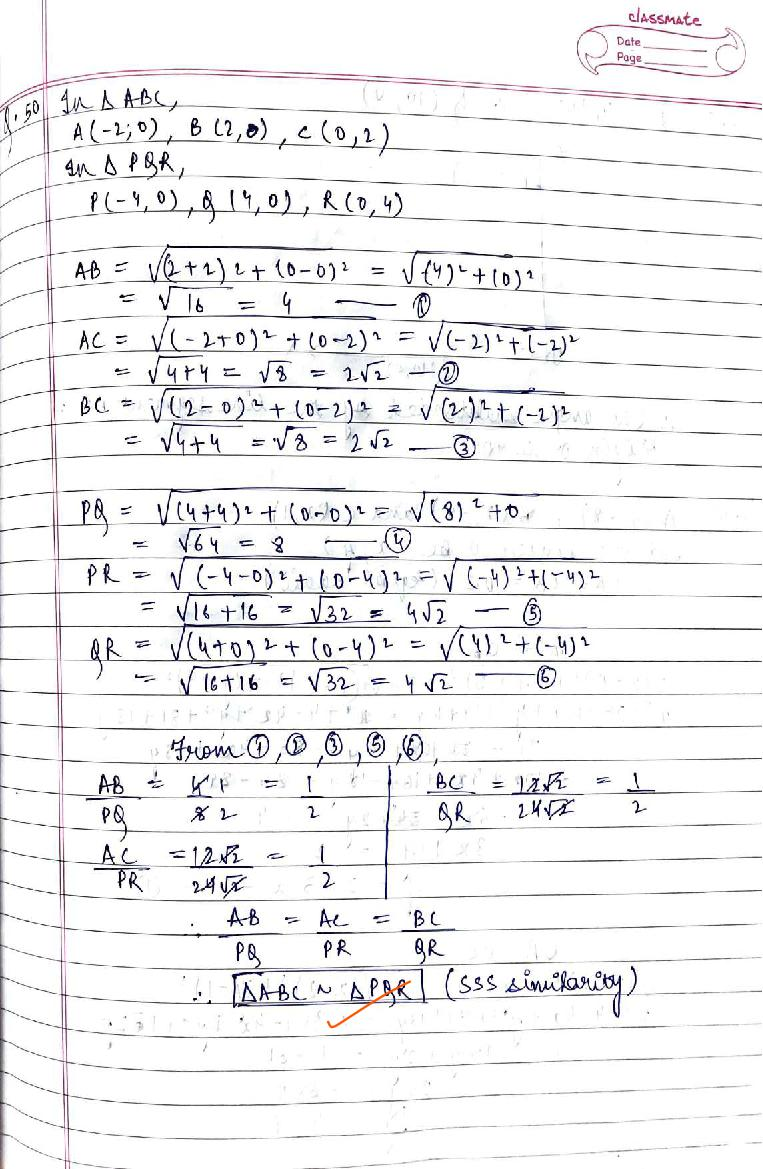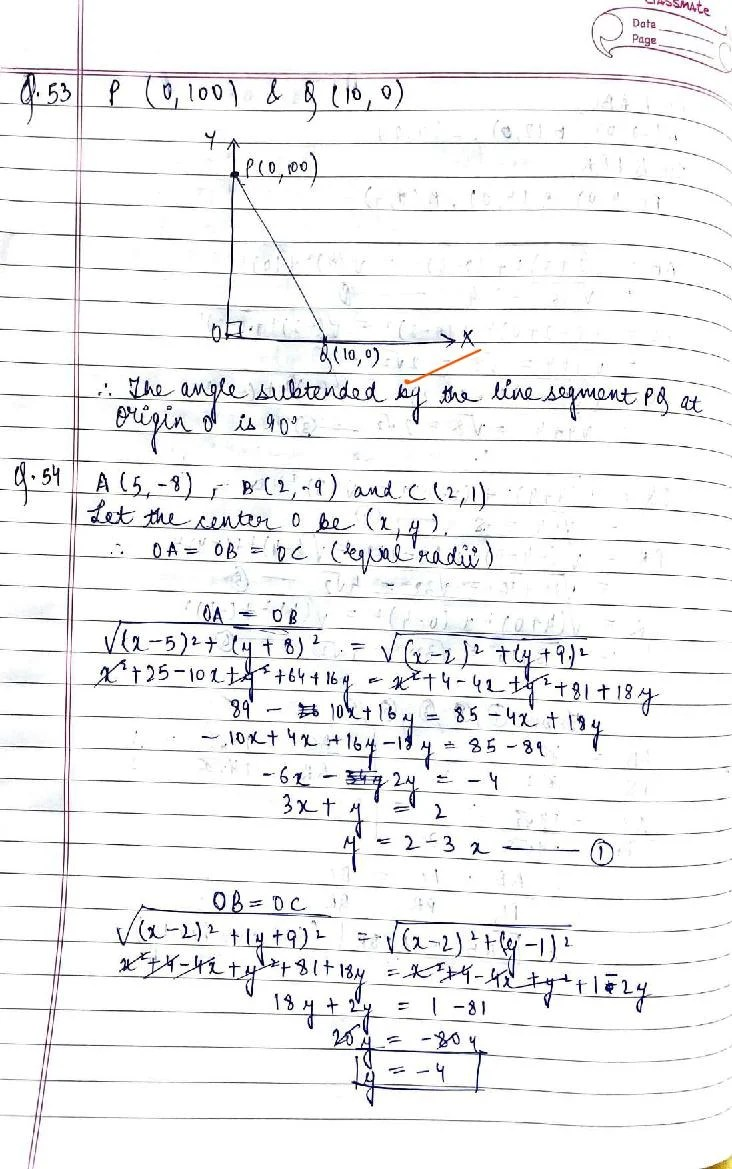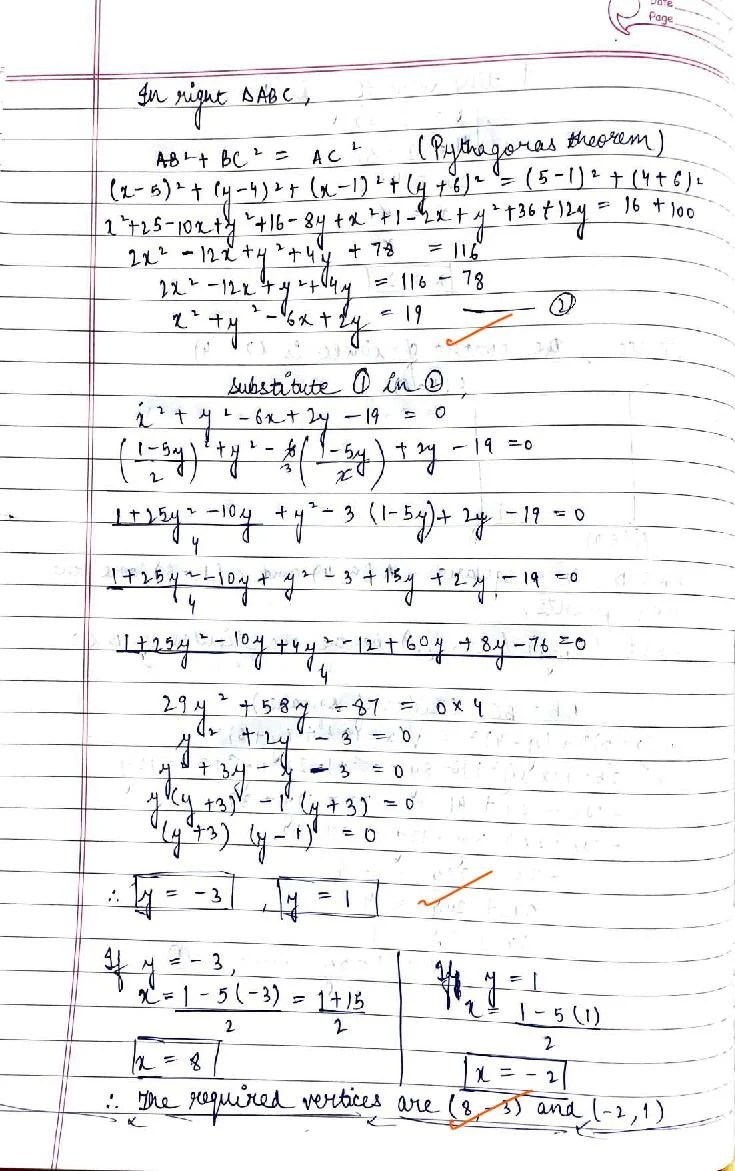# RD Sharma Solutions Class 10 Chapter 6 Coordinate Geometry Exercise 6.2

Here you can get free RD Sharma Solutions for Class 10 Maths Chapter 6 Coordinate Geometry Exercise 6.2. All RD Sharma Book Solutions are given here exercise wise for the chapter Coordinate Geometry. RD Sharma Solutions are helpful in the preparation of several school level, graduate and undergraduate level competitive exams. Practicing questions from RD Sharma Mathematics Solutions for Class 10 Chapter 6 Coordinate Geometry is proven to enhance your math skills.

 Class: Class 10th Chapter: Chapter 6 Exercise: Exercise 6.2 Name: Coordinate Geometry

## RD Sharma Solutions Class 10 Chapter 6 Coordinate Geometry Exercise 6.2

RD Sharma Class 10 Solutions Chapter 6 for Coordinate Geometry Exercise 6.2 are given below.

RD Sharma Solutions Class 10 Chapter 6 Coordinate Geometry Exercise 6.2